# Texas Go Math Grade 7 Lesson 4.2 Answer Key Using Similar Shapes

Refer to our Texas Go Math Grade 7 Answer Key Pdf to score good marks in the exams. Test yourself by practicing the problems from Texas Go Math Grade 7 Lesson 4.2 Answer Key Using Similar Shapes.

## Texas Go Math Grade 7 Lesson 4.2 Answer Key Using Similar Shapes

Example 1

△ABC ~ △JKL. Find the unknown measures.
A. Find the unknown side, x.B. Find y.
∠K corresponds to ∠B
y = ∠103°
Corresponding angles of similar triangles have equal angle measures.

Reflect

Question 1.
Analyze Relationships What other proportion could be used to find the value of x in the example? Explain.
We could use proportion between the other two sides that were not used: AC and JL and do the same calculation.
$$\frac{A C}{J L}$$ = $$\frac{B C}{K L}$$
$$\frac{16}{56}$$ = $$\frac{12}{x}$$
$$\frac{16 \div 8}{56 \div 8}=\frac{2}{7}=\frac{12}{x}$$
2x = 7 × 12
2x = 84
x = 42 cm

△ABC ~ △JGH. Find the unknown measures.

Question 2.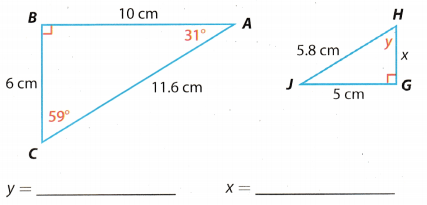∠y corresponds to ∠BCA because they are both on the right from the right angle.
∠y = ∠BCA = 59°
Write a proportion using corresponding sides.
AB corresponds to GJ, and BC corresponds to GH.
$$\frac{A B}{G J}$$ = $$\frac{B C}{G H}$$
$$\frac{10}{5}$$ = $$\frac{6}{x}$$
10x = 5 × 6
10x = 30
x = 3 cm

Question 3.∠s corresponds to ∠HJG because they are obtuse angles. A triangle can have only one obtuse angle.
∠s = ∠HJG = 120°
Write a proportion using corresponding sides
AB corresponds to GJ, and AC corresponds to HJ.Question 4.
These rectangular gardens are similar in shape. Find the width of the smaller garden.Write proportions between corresponding sides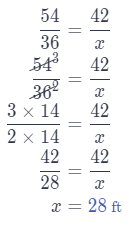Question 5.
A student who is 4 feet tall stands beside a tree. The tree has a shadow that is 12 feet long at the same time that the shadow of the student is 6 feet long. Find the height of the tree. _________
Write a proportionThe tree is 8 ft tall.

Question 6.
A photographer is taking a photo of a statue of Paul Bunyan, the legendary giant lumberjack. He measures the length of his shadow and the shadow cast by the statue. Find the height of thePaul Bunyan statue. ___________
Write a proportionThe statue of Paul Bunyan is 18 ft tall.

△ABC ~ △XYZ in each pair. Find the unknown measures (Example 1)

Question 1.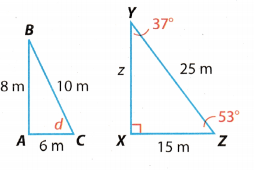∠d corresponds to ∠XZY because they are both on the right from the right angLe
∠d = ZXZY = 53°
Write a proportion using corresponding sides.
AC corresponds to XZ, and AB corresponds to XY
$$\frac{A C}{X Z}$$ = $$\frac{A B}{X Y}$$
$$\frac{6}{15}$$ = $$\frac{8}{z}$$
6z = 15 × 8
6z = 120
z = 20 m

Question 2.∠s corresponds to ∠CBA.
∠s = ∠CBA = 85°
Write a proportion using corresponding sides
AB corresponds to XY, and BC corresponds to YZ.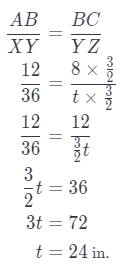Question 3.∠x corresponds to ∠ACB.
∠x = ∠ACB = 46°
Write a proportion using corresponding sides.
AC corresponds to XZ, and AB corresponds to XY.
$$\frac{X Z}{A C}$$ = $$\frac{X Y}{A B}$$
$$\frac{44}{11}$$ = $$\frac{t}{8}$$
4 = $$\frac{t}{8}$$
t = 4 × 8
t = 32 in.

Question 4.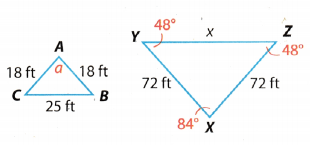∠a corresponds to ∠YXZ.
∠a = ∠YXZ = 84°
Write a proportion using corresponding sides
AC corresponds to XZ, and BC corresponds to YZ.
$$\frac{X Z}{A C}$$ = $$\frac{Y Z}{B C}$$
$$\frac{72}{18}$$ = $$\frac{x}{25}$$
x = $$\frac{x}{25}$$
x = 4 × 25
x = 100 ft

The rectangles in each pair are similar. Find the unknown measures. (Example 1)

Question 5.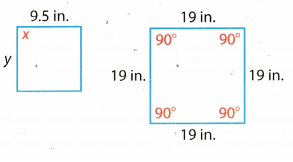Al angles in a rectangle (in this case square) are equal to 90°.
Thus, ∠x = 90°.
Since we concluded the second rectangle is a square (all sides are equal), then the first rectangle is a square too.
Thus, y = 9.5 in

Question 6.All angles in a rectangle are equal to 90°.
Thus, ∠f = 90°.
Write a proportion using corresponding sides.
RS corresponds to BC, and QR corresponds to AB.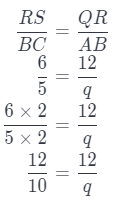q = 10 cm

Question 7.
Samantha wants to find the height of a pine tree in her yard. She measures the height of the mailbox at 3 feet and its shadow at 4.8 feet. Then she measures the shadow of the tree at 56 feet. How tall is the tree? (Example 3)
Write a proportion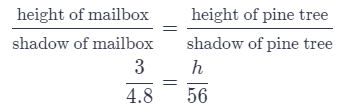4.8h = 3 × 56
4.8h = 168
h = 35 feet
The height of the pine tree is 35 feet.

Essential Question Check-In

Question 8.
Describe how you could use your height and a yardstick to determine the unknown height of a flagpole.
Then, we would write a proportion using thoes measures.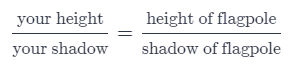Question 9.
A cactus casts a shadow that is 15 ft long. A gate nearby casts a shadow that is 5 ft long. Find the height of the cactus.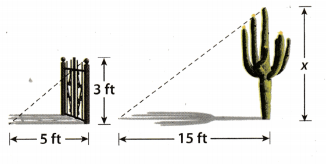Write a proportion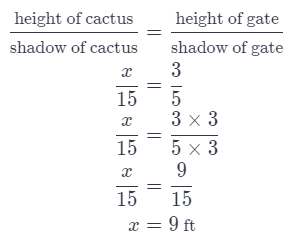The height of the cactus is 9 ft.

Question 10.
Two ramps modeled with triangles are similar. Which side of triangle KOA corresponds to $$\overline{J N}$$? Explain.We can see that $$\overline{J N}$$ Lies on the left side of the right angLe. In triangle KOA $$\overline{K O}$$ Lies on the Left side of the right angle.
Thus, $$\overline{K O}$$ responds to $$\overline{J N}$$.

Question 11.
A building with a height of 14 m casts a shadow that is 16 m long while a taller building casts a 24 m long shadow. What is the height of the taller building?
Write a proportion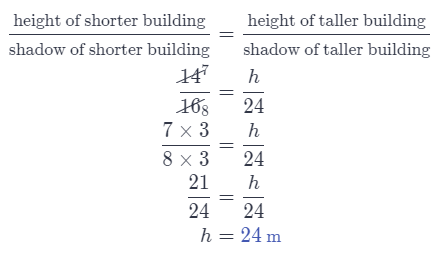The taller building is 24 m tall.

Question 12.
Katie uses a copy machine to enlarge her rectangular design that is 6 in. wide and 8 in. long. The new width is 10 in. What is the new length?
Write a proportion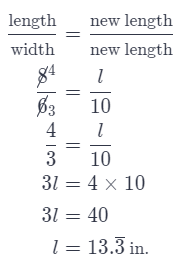New length is $$13 . \overline{3}$$ in.

Question 13.
Art An art exhibit at a local museum features several similarly shaped metal cubes welded together to make a sculpture. The smallest cube has a edge length of 6 inches.
a. What are the edge lengths of the other cubes if the ratios of similarity to the smallest cube are 1.25, $$\frac{4}{3}$$, 1.5, $$\frac{7}{4}$$, and 2 respectively?
l1 = length of the smallest cube = 6 in.
Calculate lengths of other cubes using given ratios.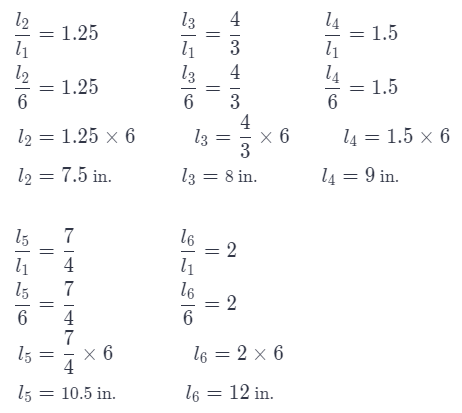b. If the artist wanted to add a smaller cube with an edge length with a ratio of $$\frac{2}{3}$$ to the sculpture, what size would the cube be?
Again, use the given ratio to calculate la
$$\frac{l_{a}}{l_{1}}$$ = $$\frac{2}{3}$$
$$\frac{l_{a}}{6}$$ = $$\frac{2}{3}$$
la = $$\frac{2}{3}$$ × 6
la = 4 in.

c. Why do you only have to find the length of one edge for each cube?
We only have to find length of one edge for each cube, because all edges of a cube are of equal length.

△XYZ ~ △PQR in each pair. Find the unknown measures.

Question 14.∠b corresponds to ∠XZY
∠d = ∠XZY = 89°
Write a proportion using corresponding sides.
XZ corresponds to PR, and YZ corresponds to RQ.
$$\frac{P R}{X Z}$$ = $$\frac{R Q}{Y Z}$$
$$\frac{20}{8}$$ = $$\frac{a}{9}$$
$$\frac{5}{2}$$ = $$\frac{a}{9}$$
2a = 5 × 9
2a = 45
a = 22.5 cm

Question 15.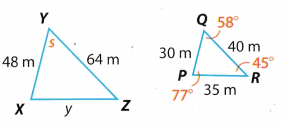∠s corresponds to ∠RQP.
∠s = ∠RQP = 58°
Write a proportion using corresponding sides.
x corresponds to and XZ corresponds to PR.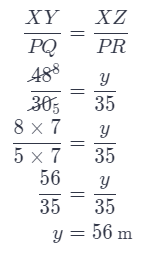Question 16.
Two common envelope sizes are 3$$\frac{1}{2}$$ in. × 6$$\frac{1}{2}$$ in. and 4in. × 9$$\frac{1}{2}$$ in. Are these envelopes similar? Explain.
Check if the ratios of the corresponding sides are equal.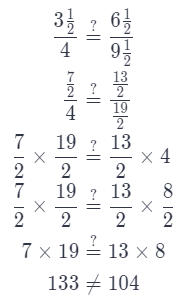Although all corresponding angles of enveLopes are equal, the ratios of corresponding sides are not equal.
Thus, the envelopes are not similar

Question 17.
A pair of rectangular baking pans come in a set together for \$15. One pan is 13 inches by 9 inches and the other pan is 6 inches by 6 inches. Without doing any calculations, how can you tell that these pans are not similar?
We can see right away that the sides of the second pan are equal. Thus, the second pan has a shape of a square white the first one has a shape of a rectangle.
From this, we can conclude that the pans are not similar.

H.O.T. Focus On Higher Order Thinking

Question 18.
Draw Conclusions In the similar triangles used in indirect measurement with the shadows of a flagpole and a person, which sides of the triangles represent the rays of the sun?
The hypotenuse represents the rays of the sun.

Question 19.
Make a Conjecture Do you think it is possible to use indirect measurement with shadows if the sun is directly overhead? Explain.
No, it is not possible.
When the sun is directly overhead, the Length of the shadow is 0. Thus, comparing any ratio including shadow side in it would equal to 0 ($$\frac{0}{x}$$), or would not be possible to divide ($$\frac{x}{0}$$)

Question 20.
Analyze Relationships Joseph’s parents have planted two gardens. One is square and has an area of 25 ft2. The other one has two sides equal to $$\frac{2}{3}$$ of one side of the square, and the other two sides equal to $$\frac{5}{2}$$ of one side of the square.

a. Find the dimensions of the other garden. Explain how you found your answer.
If first garden ¡s a square, that means his area is calculated with the following formula:
A = a2
Now, calculate its side using that A = 25 ft2.
25 = a2
a = $$\sqrt {25}$$
a = 5 ft
Next, calculate the sides of the other garden using given ratios.
x = first and third side of the other garden
y = second and fourth side of the other garden
x = $$\frac{2}{3}$$ × 5
x = $$\frac{10}{3}$$ ft

y = $$\frac{5}{2}$$ × 5
y = $$\frac{25}{2}$$ ft

b. Find the area of the other garden.
A = $$\frac{10}{3}$$ × $$\frac{25}{2}$$
A = $$\frac{5 \times 25}{3 \times 1}$$
A = $$\frac{125}{3}$$ ft2